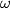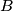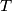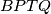### IMO Shortlist 2015 problem G4

Kvaliteta:
Avg: 0,0
Težina:
Avg: 7,0

Let$ABC$ be an acute triangle and let$M$ be the midpoint of$AC$. A circle$\omega$ passing through$B$ and$M$ meets the sides$AB$ and$BC$ at points$P$ and$Q$ respectively. Let$T$ be the point such that$BPTQ$ is a parallelogram. Suppose that$T$ lies on the circumcircle of$ABC$. Determine all possible values of$\frac{BT}{BM}$.

(Russia)

Izvor: https://www.imo-official.org/problems/IMO2015SL.pdf